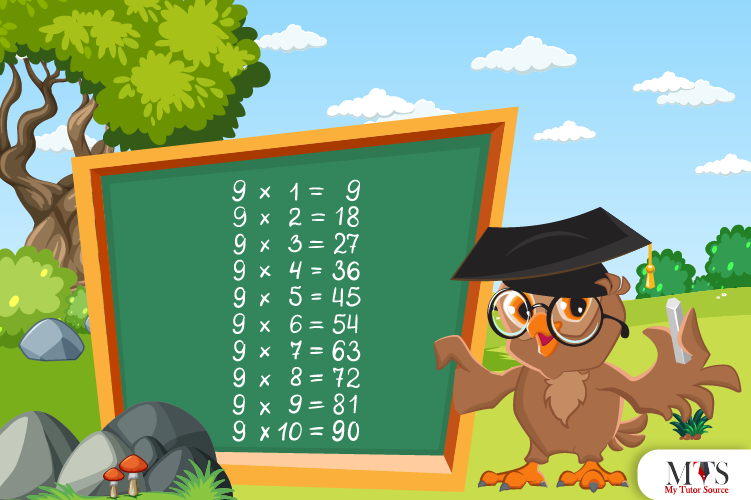# Multiplication Table of 9 – Tips to Memorize 9 Times Table & Example QuestionsStudents find memorizing 9 times table easier if they have already learned all lesser times tables. It is one of the easiest multiplication tables so far. Multiplication is a part of life and a transferable skill that helps solve GCSE level questions and beyond, even in everyday adult life. School professors and private math tutors ensure students understand and memorize the basics and multiplication tables of mathematics to do mental arithmetic and grow confident. Learning a 9 times table is important for quick calculations.

In this article, we’ve mentioned the fun tips to memorize the table of 9 along with example questions.

## Table of 9

Before learning the table of 9, let us share an interesting fact about 9. It is the most significant single-digit perfect square number and a composite number. Below we have given the 9 times table. Let us read it out aloud and write for learning purposes:

9 x 1 = 9
9 x 2 = 18
9 x 3 = 27
9 x 4 = 36
9 x 5 = 45
9 x 6 = 54
9 x 7 = 63
9 x 8 = 72
9 x 9 = 81
9 x 10 = 90
9 x 11 = 99
9 x 12 = 108
9 x 13 = 117
9 x 14 = 126
9 x 15 = 135
9 x 16 = 144
9 x 17 = 153
9 x 18 = 162
9 x 19 = 171
9 x 20 = 180

## Method to Read Table of 9

As we have read the 9 times table up to 20 mentioned-above. In this section, we have provided the table in words to teach the method of reading 9 times table.

Nine ones are nine (9)
Nine twos are eighteen (18)
Nine threes are twenty-seven (27)
Nine fours are thirty-six (36)
Nine fives are forty-five (45)
Nine sixes are fifty-four (54)
Nine sevenths are sixty-three (63)
Nine eighths are seventy-two (72)
Nine nines are eighty-one (81)
Nine tens are ninety (90)
Nine elevens are ninety-nine (99)
Nine twelves are one hundred and eight (108)
Nine thirteenths are one hundred and seventeen (117)
Nine fourteens are one hundred and twenty-six (126)
Nine fifteens are one hundred and thirty-five (135)
Nine sixteenths are one hundred and forty-four (144)
Nine seventeens are one hundred and fifty-three (153)
Nine eighteenths are one hundred and sixty-two (162)
Nine nineteen are one hundred and seventy-one (171)
Nine twenties are one hundred and eighty (180)

## Tips for Memorizing Multiplication Table of 9

Here are a few tips to help students memorize the multiplication table of 9 and less daunting tasks:

• The most exciting and easy tip students use to memorize 9 times table is the order of units,’ and tens’ digits place where tens’ place numbers increase and units’ place numbers decrease from top to bottom.
Below is the table we have designed for you as an example:
• Not all multiplication tables follow a pattern like the 9 times table does. If you reverse the product of the first 5 multiples of the 9 times table, you will get the product answer of the next 6 to 10 multipliers. This is how:

9 x 1 = 09 ⇒ 9 x 10 = 90

9 x 2 = 18 ⇒ 9 x 9 = 81

9 x 3 = 27 ⇒ 9 x 8 = 72

9 x 4 = 36 ⇒ 9 x 6 = 63

9 x 5 = 45 ⇒ 9 x 5 = 54

• Here is another informative yet exciting fact about the table of 9. To check if the product of the 9 times table is correct or not, simply sum them up. If the answer is 9, then it is undoubtedly correct. Let us see how:

### Example Question of Table of 9

Question: There are nine colors in a coloring box. How many colors are there in eleven coloring boxes?

Given that,
Total number of coloring boxes = 11
Total colors in each box = 9
Total colors in all = 11 x 9 = 99
Hence, there are 99 colors in total.

Question: Evaluate 9 times 5 plus 5 and 9 times 5 minus 5, using a 9 times table.

Solution:

Given that,
9 times 5 plus 5 and 9 times 5 minus 5
In mathematical form,
= 9 x 5 + 5 and 9 x 5 – 5
= 45 + 5 and 45 – 5
= 50 and 40
Hence, 9 times 5 plus 5 and 9 times 5 minus 5 are equal to 50 and 40, respectively.

Question: Check whether the 9 times 4 minus 10 is 26? Use a multiplication table of 9.

Solution:

Given that,
9 times 4 minus 10
In mathematical form,
= 9 x 4 – 10
= 36 – 10
= 26
Hence Proved, 9 times 4 minus 10 is 26.

Question: How many times of 9 is equal to 162?

Solution:

Let ‘a’ be the number
⇒ a x 9 = 162
⇒ a = 162/9
⇒ a = 18
Therefore, 18 times 9 is equal to 162.

Question: Each bag has 5 books. How many books are there in 9 such bags? (Use repeated addition method)

Solution:

Total number of books = 9 x 5 = 54
Total number of books = 9 + 9 + 9 +9 + 9 = 54
Thus, there are 54 books in total in 9 bags.

### Find Top Tutors in Your AreaWith over 3 years of experience in teaching, Chloe is very deeply connected with the topics that talk about the educational and general aspects of a student's life. Her writing has been very helpful for students to gain a better understanding of their academics and personal well-being. I’m also open to any suggestions that you might have! Please reach out to me at chloedaniel402 [at] gmail.com